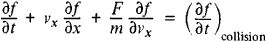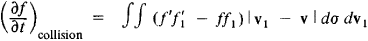# Boltzmann equation

Also found in: Dictionary, Thesaurus, Medical, Wikipedia.

## Boltzmann equation

An equation derived by the Austrian physicist Ludwig Boltzmann in the 1870s that shows how the distribution of molecules, atoms, or ions in their various energy levels depends on the temperature of the system; the system is in thermal equilibrium, with excitation balanced by de-excitation. The equation gives the ratio of the number density (number per unit volume) of molecules, atoms, or ions, N 2, in one energy level to the number density, N 1, in another lower energy level as
N 2 /N 1 = (g 2 /g 1 )eE/kT

g 1 and g 2 are the degeneracies of the two levels, i.e. the multiplicity of energy levels with the same energy, E is the energy required to excite the molecule, atom, or ion from the lower to the higher energy level, k is the Boltzmann constant, and T is the thermodynamic temperature. Thus as T increases a greater number of species will become excited.

The Boltzmann equation together with the Saha ionization equation are widely used to interpret the absorption and emission spectra of stars and to determine stellar temperatures and densities.

Collins Dictionary of Astronomy © Market House Books Ltd, 2006
The following article is from The Great Soviet Encyclopedia (1979). It might be outdated or ideologically biased.

## Boltzmann (Kinetic) Equation

an equation for the distribution function f(v, r, t) of gas molecules in the velocities ν and coordinates r (as functions of time t) that describes nonequilibrium processes in gases of low density. The function f determines the average number of particles having velocities within a small range from ν to ν + Δν and coordinates within a small range from r to r + Δr. If the distribution function depends only on the coordinate x and the velocity component vx, then the Boltzmann (kinetic) equation has the formwhere m is the mass of the particle. The rate of change of the distribution function is characterized by the partial derivative ∂f/∂t. The second term in the equation, which is proportional to the partial derivative of the distribution function with respect to the coordinate, takes account of the change in f as a result of the movement of particles in space. The third term determines the change in the distribution function owing to the action of external forces F. The term on the right-hand side of the equation, which characterizes the rate of change of the distribution function owing to collisions between particles, depends on f and the nature of the forces of interaction between particles and is equal toHere, f, f1, and fʹ, f1ʹ are the distribution functions of the molecules before and after collision, respectively; v, v1 are the velocities of the molecules before collision; and dσ =σdΩ is the differential effective scattering cross section into the solid angle dΩ (in the laboratory coordinate system) dependent on the law of molecular interaction. When molecules are simulated by rigid elastic spheres (of radius R), σ = 4R2 cos θ, where θ is the angle between the relative velocity v1 - v of the colliding molecules and the line connecting its centers. The kinetic equation was derived by L. Boltzmann in 1872.

Various generalizations of the Boltzmann kinetic equation describe the behavior of electron gas in metals, of phonons in crystal lattices, and so forth (however, these equations are often called simply kinetic equations, or transport equations).

G. IA. MIAKISHEV

References in periodicals archive ?
Simonella, "On the validity of the Boltzmann equation for short range potentials," Reviews in Mathematical Physics, vol.
Yang, "Regularity of solutions to the spatially homogeneous Boltzmann equation without angular cutoff," Discrete and Continuous Dynamical Systems A, vol.
These glancing collisions turn out to be dominant type of collision for the full Boltzmann equation with long-range interactions.
The discrete Boltzmann equation (1) for the distribution function [f.sub.i] [Equivalent to] [f.sub.i] ([x.sub.[alpha]], t) can be solved numerically using an appropriate finite difference scheme, and complicated flows in the generalized coordinate system can be simulated in a simple algorithm using a coordinate transformation technique.
That last step was "really a breakthrough from my point of view," comments Roberto Benzi of the University of Rome Tor Vergata, a pioneer in the use of the Boltzmann equation for fluid flows.
First there were the grave difficulties incumbent on trying to reconcile the reversible, recurrent dynamics of the micro-components with the irreversible, monotonic approach to equilibrium described by the Boltzmann equation and the H-Theorem.
A discretization of the Boltzmann equation in time and space leads to the lattice Boltzmann equation [20, 21]:
However, the most notable example is perhaps provided by dilute hard-sphere systems ("gases") characterized by a large number of particles (N [equivalent to] 1/[epsilon] >> 1) and a small (i.e., infinitesimal) diameter [sigma] ~ O([[epsilon].sup.1/2]) of the same hard-spheres, for which the Boltzmann equation applies.
LBM describes the behavior of a collection of particles, and instead of macroscopic equation of fluid dynamics, it is based on the Boltzmann equation which describes dynamics of a gas on a mesoscopic scale :
Reduced from the collisional Boltzmann equation, the mandatory set of the Vlasov-Maxwell equations for ions and electrons are expressed jointly as follows: (15,35)

Site: Follow: Share:
Open / Close